### 乾貨：可視化項目實戰經驗分享，輕鬆玩轉Bokeh（建議收藏）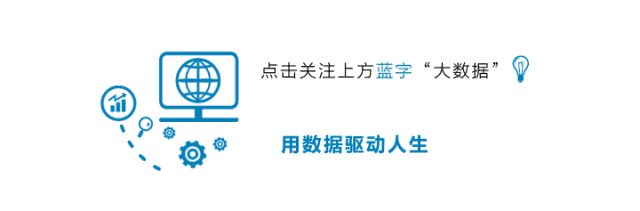1. Bokeh 基礎介紹

2. 在 Bokeh 中添加主動交互功能

3. 在 Bokeh 中創建交互式可視化應用程序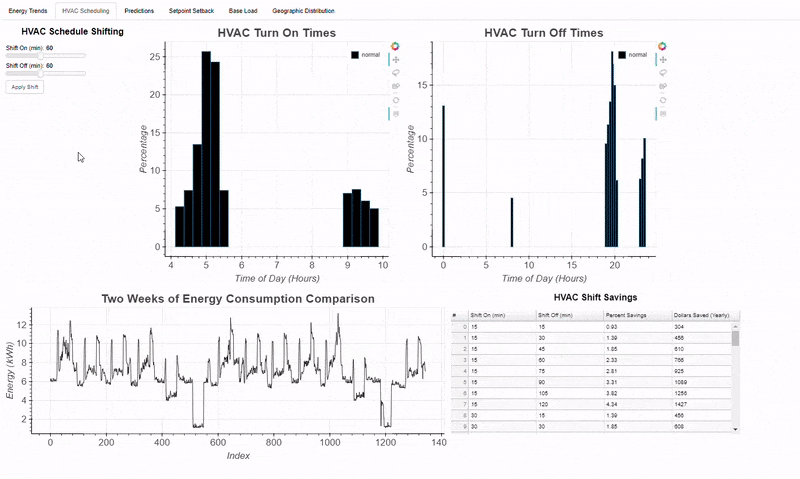## 01 Bokeh 基礎

Bokeh 的主要概念是圖形一次構建一層。我們首先創建一個圖形(figure)，然後在圖形中添加稱為圖形符號(glyphs)的元素。glyphs 可以根據所需的用途呈現多種形狀：圓形(circles)，線條(lines)，補丁(patches)，條形(bars)，弧形(arcs)等。

``# bokeh basicsfrom bokeh.plotting import figurefrom bokeh.io import show, output_notebook# Create a blank figure with labelsp = figure(plot_width = 600, plot_height = 600,            title = 'Example Glyphs',           x_axis_label = 'X', y_axis_label = 'Y')# Example datasquares_x = [1, 3, 4, 5, 8]squares_y = [8, 7, 3, 1, 10]circles_x = [9, 12, 4, 3, 15]circles_y = [8, 4, 11, 6, 10]# Add squares glyphp.square(squares_x, squares_y, size = 12, color = 'navy', alpha = 0.6)# Add circle glyphp.circle(circles_x, circles_y, size = 12, color = 'red')# Set to output the plot in the notebookoutput_notebook()# Show the plotshow(p)``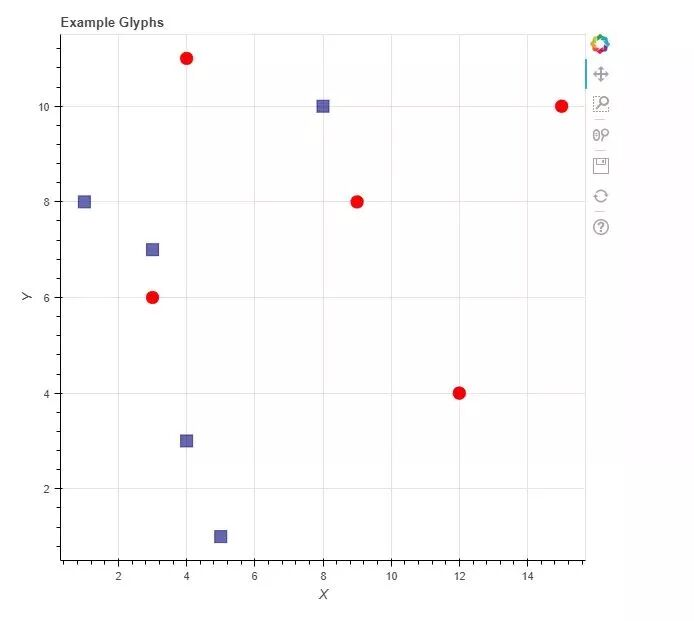``# Read the data from a csv into a dataframeflights = pd.read_csv('../data/flights.csv', index_col=0)# Summary stats for the column of interestflights['arr_delay'].describe()out[]:count    327346.000000mean          6.895377std          44.633292min         -86.00000025%         -17.00000050%          -5.00000075%          14.000000max        1272.000000``

``"""Bins will be five minutes in width, so the number of bins is (length of interval / 5). Limit delays to [-60, +120] minutes using the range."""arr_hist, edges = np.histogram(flights['arr_delay'],                                bins = int(180/5),                                range = [-60, 120])# Put the information in a dataframedelays = pd.DataFrame({'arr_delay': arr_hist,                        'left': edges[:-1],                        'right': edges[1:]})``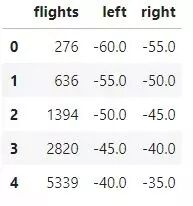flights 列是從 left 到 right 的每個延遲間隔內的航班數量。從這裏開始，我們可以創建一個新的 Bokeh 圖形，並添加一個指定適當參數的 quad

``# Create the blank plotp = figure(plot_height = 600, plot_width = 600,            title = 'Histogram of Arrival Delays',           x_axis_label = 'Delay (min)]',            y_axis_label = 'Number of Flights')# Add a quad glyphp.quad(bottom=0, top=delays['flights'],        left=delays['left'], right=delays['right'],        fill_color='red', line_color='black')# Show the plotshow(p)``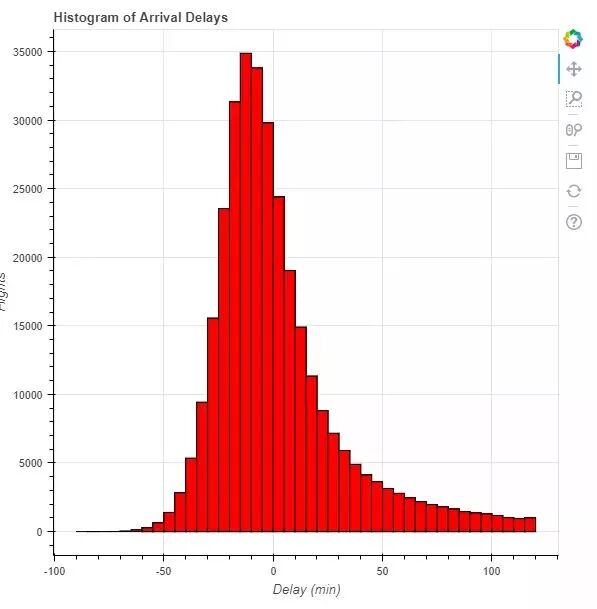### 1. 增加交互性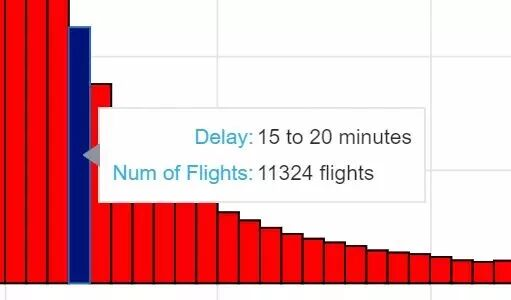``# Import the ColumnDataSource classfrom bokeh.models import ColumnDataSource# Convert dataframe to column data sourcesrc = ColumnDataSource(delays)src.data.keys()out:dict_keys(['flights', 'left', 'right', 'index'])``

``# Add a quad glyph with source this timep.quad(source = src, bottom=0, top='flights',        left='left', right='right',        fill_color='red', line_color='black')``

### 2. Bokeh 中的 HoverTool

HoverTool 的語法起初可能看起來有些複雜，但通過練習它們很容易創建。

``# Hover tool referring to our own data field using @ and# a position on the graph using \$h = HoverTool(tooltips = [('Delay Interval Left ', '@left'),                          ('(x,y)', '(\$x, \$y)')])``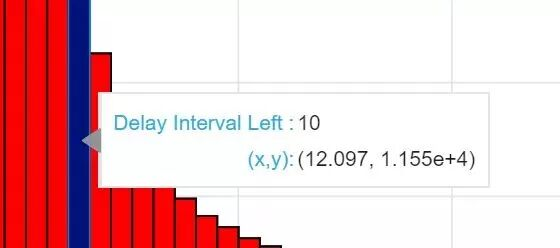（x，y）位置是圖表上鼠標的位置，對我們的直方圖不是很有幫助，因為我們要找到給定條形中對應於條形頂部的航班數量。

``# Add a column showing the extent of each intervaldelays['f_interval'] = ['%d to %d minutes' % (left, right) for left, right in zip(delays['left'], delays['right'])]``

``# Create the blank plotp = figure(plot_height = 600, plot_width = 600,            title = 'Histogram of Arrival Delays',           x_axis_label = 'Delay (min)]',            y_axis_label = 'Number of Flights')# Add a quad glyph with source this timep.quad(bottom=0, top='flights', left='left', right='right', source=src,       fill_color='red', line_color='black', fill_alpha = 0.75,       hover_fill_alpha = 1.0, hover_fill_color = 'navy')# Add a hover tool referring to the formatted columnshover = HoverTool(tooltips = [('Delay', '@f_interval'),                             ('Num of Flights', '@f_flights')])# Style the plotp = style(p)# Add the hover tool to the graphp.add_tools(hover)# Show the plotshow(p)``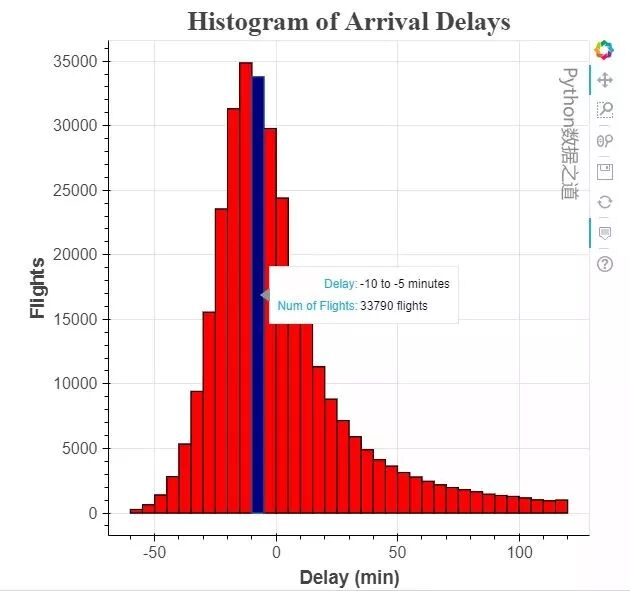``# Import savings functionfrom bokeh.io import output_file# Specify the output file and saveoutput_file('hist.html')show(p)``

## 02 在 Bokeh 中添加主動交互

Bokeh中有兩類交互：被動交互和主動交互。前面介紹的被動交互也稱為檢查器（inspectors），因為它們允許用户更詳細地查閲圖表中的信息，但不會更改顯示的信息。一個示例是當用户將鼠標懸停在數據點上時顯示的提示信息，如下：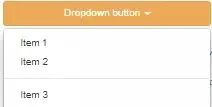### 1. 主動互動的實現方法

• make_dataset(): 按特定格式整理要顯示的特定數據

• make_plot(): 使用指定的數據繪圖

• update(): 根據用户選擇更新繪圖

#### 2. 整理數據

1. 航空公司 （在代碼中稱為 carriers）

2. 延遲的時間範圍，比如： -60 至 +120 分鐘

3. 直方圖的寬度（即 bin 大小），默認值為 5 分鐘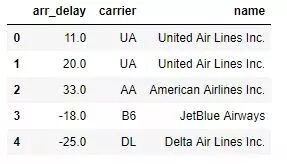``def make_dataset(carrier_list, range_start = -60, range_end = 120, bin_width = 5):    # Check to make sure the start is less than the end!    assert range_start < range_end, "Start must be less than end!"    by_carrier = pd.DataFrame(columns=['proportion', 'left', 'right',                                       'f_proportion', 'f_interval',                                       'name', 'color'])    range_extent = range_end - range_start    # Iterate through all the carriers    for i, carrier_name in enumerate(carrier_list):        # Subset to the carrier        subset = flights[flights['name'] == carrier_name]        # Create a histogram with specified bins and range        arr_hist, edges = np.histogram(subset['arr_delay'],                                       bins = int(range_extent / bin_width),                                       range = [range_start, range_end])        # Divide the counts by the total to get a proportion and create df        arr_df = pd.DataFrame({'proportion': arr_hist / np.sum(arr_hist),                               'left': edges[:-1], 'right': edges[1:] })        # Format the proportion        arr_df['f_proportion'] = ['%0.5f' % proportion for proportion in arr_df['proportion']]        # Format the interval        arr_df['f_interval'] = ['%d to %d minutes' % (left, right) for left,                                 right in zip(arr_df['left'], arr_df['right'])]        # Assign the carrier for labels        arr_df['name'] = carrier_name        # Color each carrier differently        arr_df['color'] = Category20_16[i]        # Add to the overall dataframe        by_carrier = by_carrier.append(arr_df)    # Overall dataframe    by_carrier = by_carrier.sort_values(['name', 'left'])      # Convert dataframe to column data source    return ColumnDataSource(by_carrier)``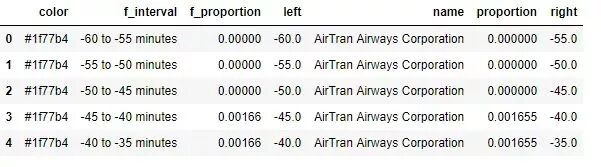``def make_plot(src):        # Blank plot with correct labels        p = figure(plot_width = 700, plot_height = 700,                  title = 'Histogram of Arrival Delays by Carrier',                  x_axis_label = 'Delay (min)', y_axis_label = 'Proportion')        # Quad glyphs to create a histogram        p.quad(source = src, bottom = 0, top = 'proportion', left = 'left', right = 'right',               color = 'color', fill_alpha = 0.7, hover_fill_color = 'color', legend = 'name',               hover_fill_alpha = 1.0, line_color = 'black')        # Hover tool with vline mode        hover = HoverTool(tooltips=[('Carrier', '@name'),                                    ('Delay', '@f_interval'),                                    ('Proportion', '@f_proportion')],                          mode='vline')        p.add_tools(hover)        # Styling        p = style(p)        return p``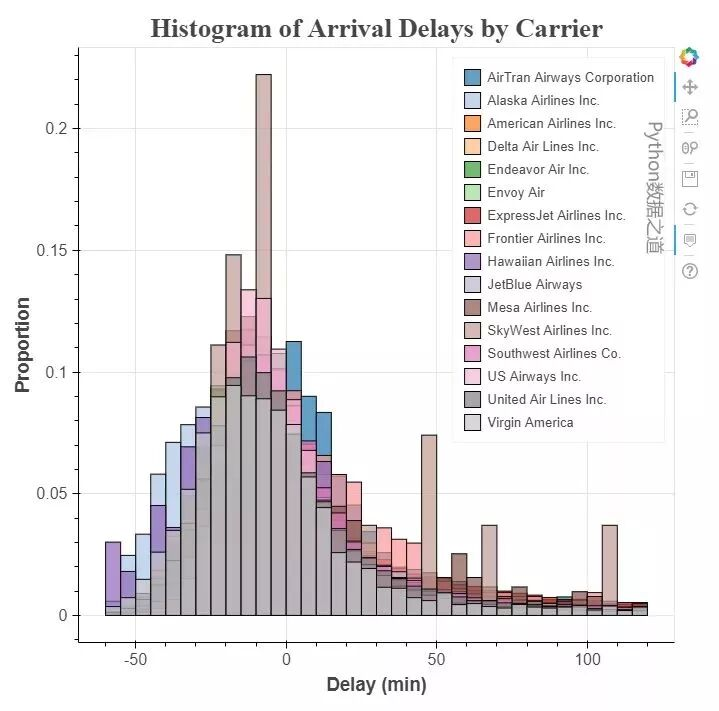• #### 創建交互的小部件

``from bokeh.models.widgets import CheckboxGroup# Create the checkbox selection element, available carriers is a  # list of all airlines in the datacarrier_selection = CheckboxGroup(labels=available_carriers,                                  active = [0, 1])``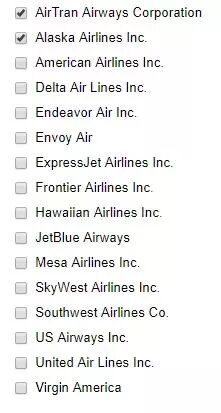Bokeh 複選框中的標籤必須是字符串，而活動值是整數。 這意味着在圖形中 'AirTran Airways Corporation' 對應數字 0 ，'Alaska Airlines Inc.' 對應數值 1。 當想要將所選複選框與航空公司匹配時，需要確保查找與所選整數活動值關聯的字符串名稱。 我們可以使用小部件的 .labels 和 .active 屬性來做到這一點：

``# Select the airlines names from the selection values[carrier_selection.labels[i] for i in carrier_selection.active]out:['AirTran Airways Corporation', 'Alaska Airlines Inc.']``

``# Update function takes three default parametersdef update(attr, old, new):    # Get the list of carriers for the graph    carriers_to_plot = [carrier_selection.labels[i] for i in carrier_selection.active]    # Make a new dataset based on the selected carriers and the     # make_dataset function defined earlier    new_src = make_dataset(carriers_to_plot,                           range_start = -60,                           range_end = 120,                           bin_width = 5)    # Update the source used in the quad glpyhs    src.data.update(new_src.data)``

``# Link a change in selected buttons to the update functioncarrier_selection.on_change('active', update)``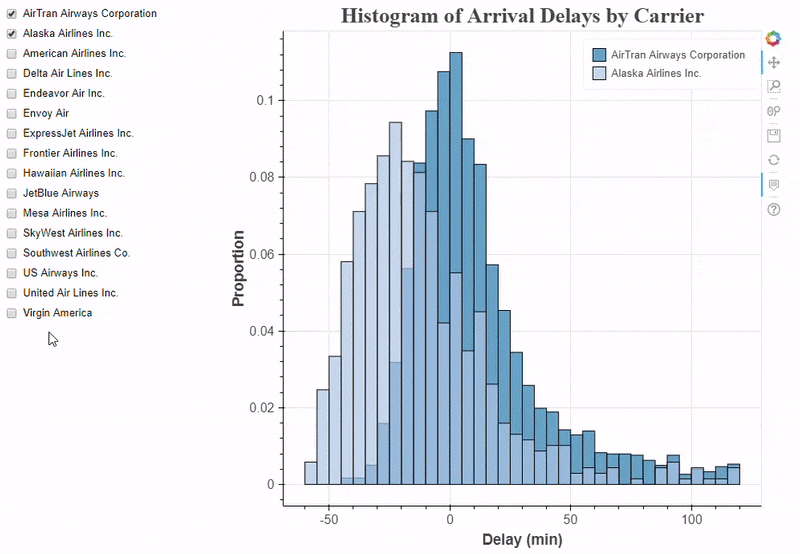2. 更多的交互式控制

``# Slider to select the binwidth, value is selected numberbinwidth_select = Slider(start = 1, end = 30,                      step = 1, value = 5,                     title = 'Delay Width (min)')# Update the plot when the value is changedbinwidth_select.on_change('value', update)# RangeSlider to change the maximum and minimum values on histogramrange_select = RangeSlider(start = -60, end = 180, value = (-60, 120),                           step = 5, title = 'Delay Range (min)')# Update the plot when the value is changedrange_select.on_change('value', update)# Update function that accounts for all 3 controlsdef update(attr, old, new):    # Find the selected carriers    carriers_to_plot = [carrier_selection.labels[i] for i in carrier_selection.active]    # Change binwidth to selected value    bin_width = binwidth_select.value    # Value for the range slider is a tuple (start, end)    range_start = range_select.value    range_end = range_select.value    # Create new ColumnDataSource    new_src = make_dataset(carriers_to_plot,                           range_start = range_start,                           range_end = range_end,                           bin_width = bin_width)    # Update the data on the plot    src.data.update(new_src.data)``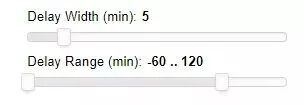``# Change plot title to match selectionbin_width = binwidth_select.valuep.title.text = 'Delays with %d Minute Bin Width' % bin_width``

### 3. 把它們放在一起

``from bokeh.layouts import column, row, WidgetBoxfrom bokeh.models import Panelfrom bokeh.models.widgets import Tabs# Put controls in a single elementcontrols = WidgetBox(carrier_selection, binwidth_select, range_select)# Create a row layoutlayout = row(controls, p)# Make a tab with the layout tab = Panel(child=layout, title = 'Delay Histogram')tabs = Tabs(tabs=[tab])``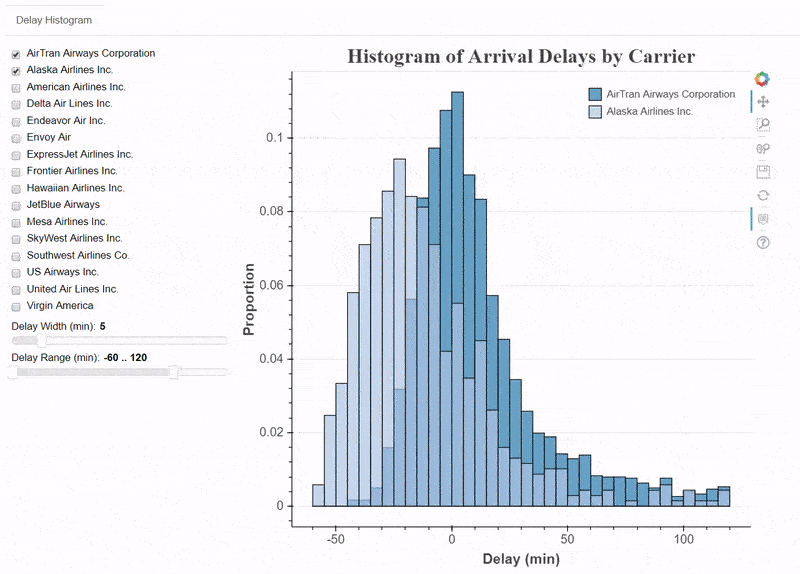03 在 Bokeh 中創建交互式可視化應用程序

### 2. Bokeh 應用程序的文件結構

``bokeh_app|+--- data|   +--- info.csv|   +--- info2.csv|+--- scripts|   +--- plot.py|   +--- plot2.py|+--- main.py``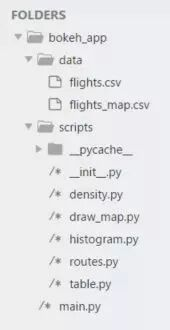### 3. 主程序文件 （main.py）

main.py 腳本就像一個 Bokeh 應用程序的執行程序。 它加載數據，將其傳遞給其他腳本，返回結果圖，並將它們組織到一個顯示中。 這將是我完整展示的唯一腳本，因為它對應用程序尤其重要。

``# Pandas for data managementimport pandas as pd# os methods for manipulating pathsfrom os.path import dirname, join# Bokeh basics from bokeh.io import curdocfrom bokeh.models.widgets import Tabs# Each tab is drawn by one scriptfrom scripts.histogram import histogram_tabfrom scripts.density import density_tabfrom scripts.table import table_tabfrom scripts.draw_map import map_tabfrom scripts.routes import route_tab# Using included state data from Bokeh for mapfrom bokeh.sampledata.us_states import data as states# Read data into dataframesflights = pd.read_csv(join(dirname(__file__), 'data', 'flights.csv'),                                               index_col=0).dropna()# Formatted Flight Delay Data for mapmap_data = pd.read_csv(join(dirname(__file__), 'data', 'flights_map.csv'),                            header=[0,1], index_col=0)# Create each of the tabstab1 = histogram_tab(flights)tab2 = density_tab(flights)tab3 = table_tab(flights)tab4 = map_tab(map_data, states)tab5 = route_tb(flights)# Put all the tabs into one applicationtabs = Tabs(tabs = [tab1, tab2, tab3, tab4, tab5])# Put the tabs in the current document for displaycurdoc().add_root(tabs)``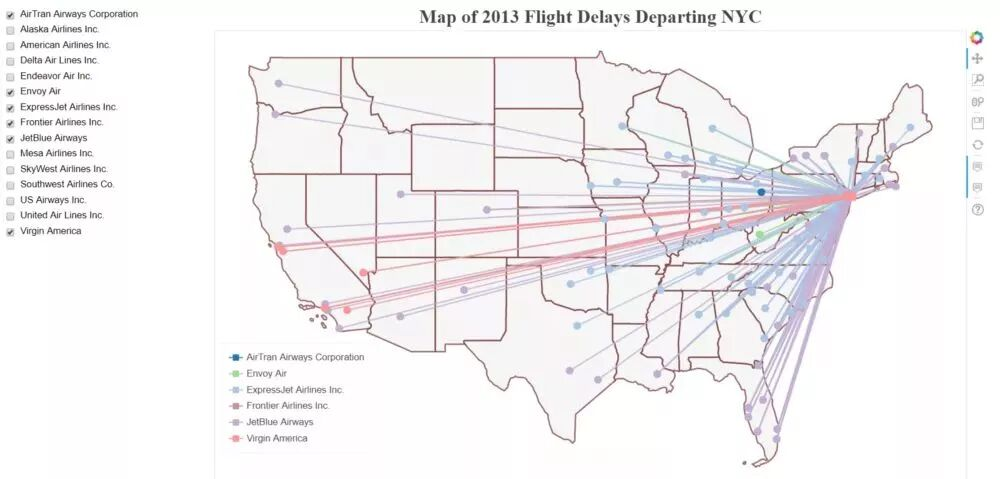``def map_tab(map_data, states):    ...    def make_dataset(airline_list):    ...       return new_src    def make_plot(src):    ...       return p   def update(attr, old, new):   ...      new_src = make_dataset(airline_list)      src.data.update(new_src.data)   controls = ...   tab = Panel(child = layout, title = 'Flight Map')   return tab``

``# Put all the tabs into one applicationtabs = Tabs(tabs = [tab1, tab2, tab3, tab4, tab5])# Put the tabs in the current document for displaycurdoc().add_root(tabs)``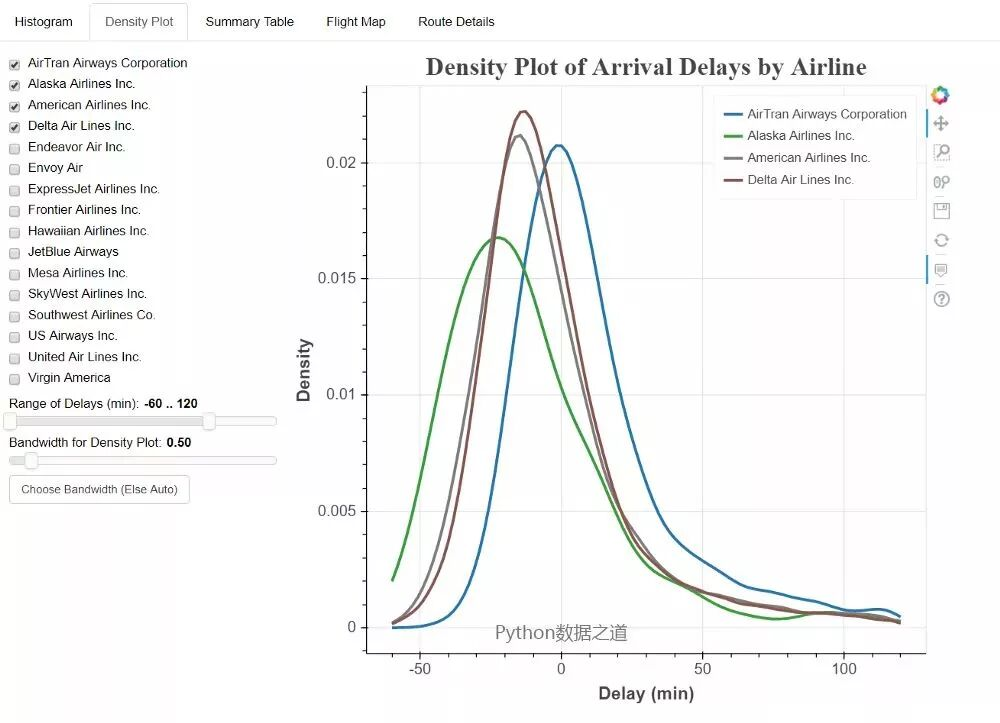### 4. 運行 Bokeh 服務器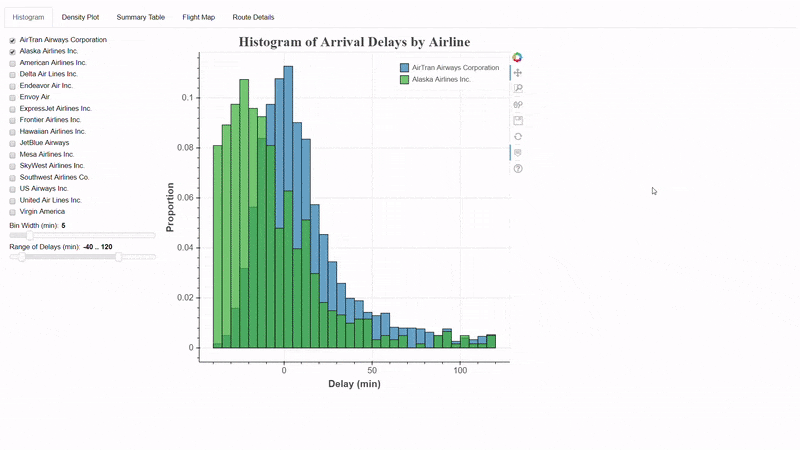5. 在 Jupyter Notebook 中進行調試

### 04 總結

1. 在開始數據科學任務（Bokeh 或其他任何東西）之前，擁有適當的框架/結構至關重要。 這樣，你就不會發現自己迷失在試圖查找錯誤的代碼的泥潭中。 此外，一旦我們開發出一個有效的框架，它可以用最少的努力重複使用。

2. 找到一個允許您快速迭代思路的調試工具至關重要。 編寫代碼 - 查看結果 - 修復錯誤，這種循環在 Jupyter Notebook 可以實現高效的開發（尤其是對於小規模項目）。

3. Bokeh 中的交互式應用程序將提升您的項目並鼓勵用户參與。 儀表板可以是一個獨立的探索項目，或突出您已經完成的所有艱難的分析工作！

4. 估計你永遠不知道在哪裏可以找到你將在工作或輔助項目中使用的下一個工具。 所以，不要害怕嘗試新的軟件和技術！

Data Visualization with Bokeh in Python, Part I:

Getting Started https://towardsdatascience.com/data-visualization-with-bokeh-in-python-part-one-getting-started-a11655a467d4

Data Visualization with Bokeh in Python, Part II:

Interactions https://towardsdatascience.com/data-visualization-with-bokeh-in-python-part-ii-interactions-a4cf994e2512

Data Visualization with Bokeh in Python, Part III:

Making a Complete Dashboard https://towardsdatascience.com/data-visualization-with-bokeh-in-python-part-iii-a-complete-dashboard-dc6a86aa6e23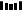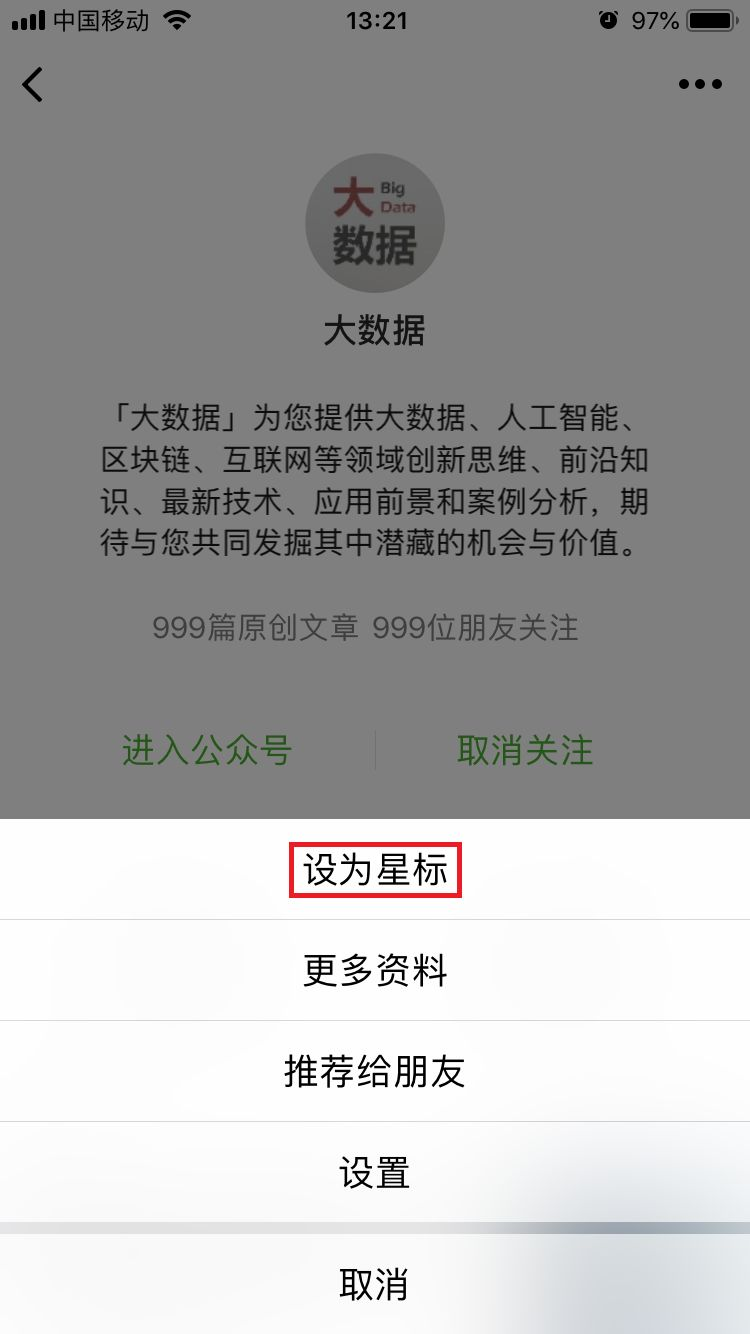PPT | 報告 | 讀書 | 書單 | 乾貨

AI | 1024 | 段子 | 區塊鏈 | 數學

• 女神節，來聊聊這幾位神一般的“程序媛”

• 馬化騰提議加強基礎科學研究，中國“芯痛”能解決嗎？

• 數據又多又散，“孤島困境”怎樣破局？

• 從數據角度看，每家公司只有2種角色：看看你更適合哪一種？

Q: 關於Bokeh你還有哪些使用技巧？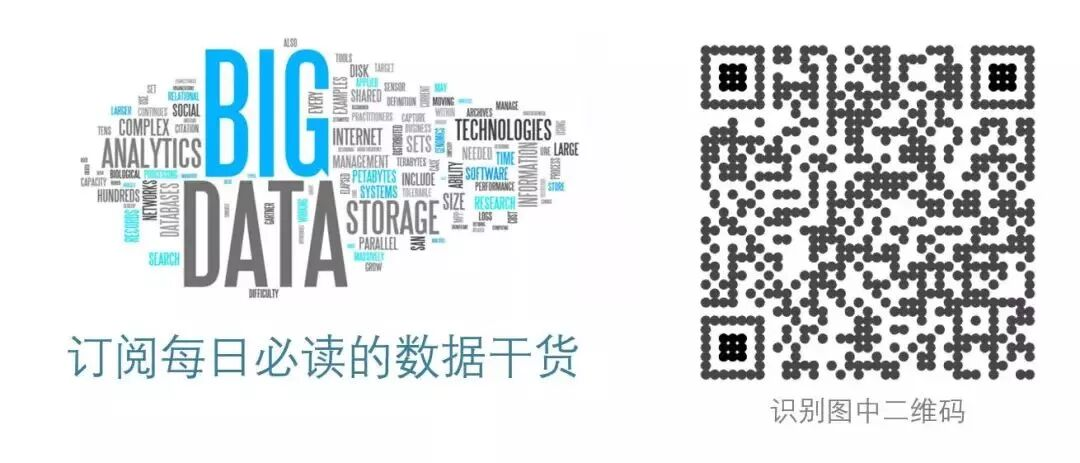https://hk.wxwenku.com/d/110021246